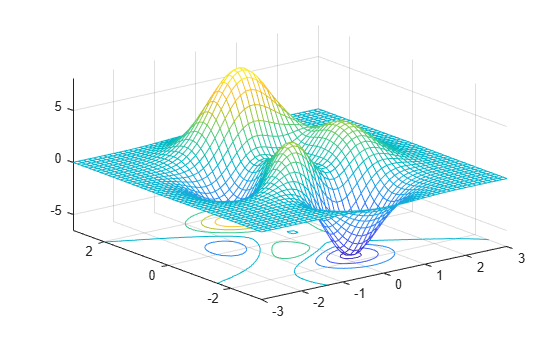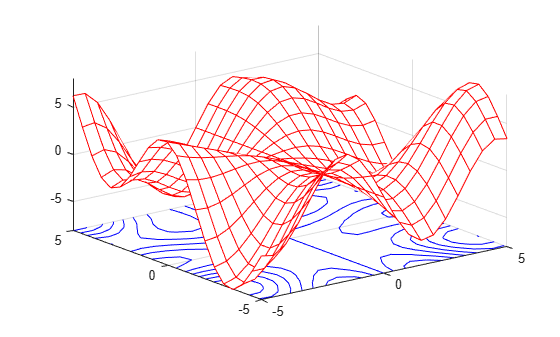Documentation

# meshc

Contour plot under mesh surface plot

## Syntax

``meshc(X,Y,Z)``
``meshc(Z)``
``meshc(___,C)``
``meshc(ax,___)``
``sc = meshc(___)``

## Description

example

````meshc(X,Y,Z)` creates a mesh plot with a contour plot underneath. A mesh plot is a three-dimensional surface that has solid edge colors and no face colors. The function plots the values in matrix `Z` as heights above a grid in the x-y plane defined by `X` and `Y`. The edge colors vary according to the heights specified by `Z`.```
````meshc(Z)` creates a mesh and contour plot and uses the column and row indices of the elements in `Z` as the x- and y-coordinates.```

example

````meshc(___,C)` additionally specifies the color of the edges.```
````meshc(ax,___)` plots into the axes specified by `ax` instead of the current axes. Specify the axes as the first input argument.```

example

````sc = meshc(___)` returns a graphics array that includes the chart surface object and the contour object. Use `sc` to modify the mesh and contour plots after they are created. For a list of properties, see Surface Properties and Contour Properties.```

## Examples

collapse all

Create three matrices of the same size. Then plot them as a mesh plot with a contour plot underneath. The mesh plot uses `Z` for both height and color.

```[X,Y] = meshgrid(-3:.125:3); Z = peaks(X,Y); meshc(X,Y,Z)```Specify the colors for a mesh and contour plot by including a fourth matrix input, `C`. The plots use `Z` for height and `C` for color. Specify the colors using a colormap, which uses single numbers to stand for colors on a spectrum. When you use a colormap, `C` is the same size as `Z`. Add a color bar to the graph to show how the data values in `C` correspond to the colors in the colormap.

```[X,Y] = meshgrid(-3:.125:3); Z = peaks(X,Y); C = X.*Y; meshc(X,Y,Z,C) colorbar```Create a mesh plot with a contour plot underneath it. To allow further modifications, assign the graphics array containing the surface and contour objects to the variable `sc`.

```[X,Y] = meshgrid(-5:.5:5); Z = Y.*sin(X) - X.*cos(Y); sc = meshc(X,Y,Z);```Index into `sc` to access and modify properties of the mesh and contour plots after they are created. The mesh plot is accessible as `sc(1)` and the contour plot as `sc(2)`. For example, change the edge colors of the two plots by setting the `EdgeColor` properties.

```sc(1).EdgeColor = 'r'; sc(2).EdgeColor = 'b';```## Input Arguments

collapse all

x-coordinates, specified as a matrix the same size as `Z`, or as a vector with length `n`, where `[m,n] = size(Z)`. If you do not specify values for `X` and `Y`, `meshc` uses the vectors `(1:n)` and `(1:m)`.

When `X` is a matrix, the values must be strictly increasing or decreasing along one dimension and remain constant along the other dimension. The dimension that varies must be the opposite of the dimension that varies in `Y`. You can use the `meshgrid` function to create `X` and `Y` matrices.

When `X` is a vector, the values must be strictly increasing or decreasing.

The `XData` properties of the surface and contour objects store the x-coordinates.

Example: `X = 1:10`

Example: `X = [1 2 3; 1 2 3; 1 2 3]`

Example: `[X,Y] = meshgrid(-5:0.5:5)`

Data Types: `single` | `double` | `int8` | `int16` | `int32` | `int64` | `uint8` | `uint16` | `uint32` | `uint64` | `categorical`

y-coordinates, specified as a matrix the same size as `Z` or as a vector with length `m`, where `[m,n] = size(Z)`. If you do not specify values for `X` and `Y`, `meshc` uses the vectors `(1:n)` and `(1:m)`.

When `Y` is a matrix, the values must be strictly increasing or decreasing along one dimension and remain constant along the other dimension. The dimension that varies must be the opposite of the dimension that varies in `X`. You can use the `meshgrid` function to create `X` and `Y` matrices.

When `Y` is a vector, the values must be strictly increasing or decreasing.

The `YData` properties of the surface and contour objects store the y-coordinates.

Example: `Y = 1:10`

Example: `Y = [1 1 1; 2 2 2; 3 3 3]`

Example: `[X,Y] = meshgrid(-5:0.5:5)`

Data Types: `single` | `double` | `int8` | `int16` | `int32` | `int64` | `uint8` | `uint16` | `uint32` | `uint64` | `categorical`

z-coordinates, specified as a matrix. `Z` must have at least two rows and two columns.

`Z` specifies the height of the mesh plot at each x-y-coordinate. If you do not specify the colors, then `Z` also specifies the mesh edge colors.

The `ZData` properties of the surface and contour objects store the z-coordinates.

Example: `Z = [1 2 3; 4 5 6]`

Example: `Z = sin(x) + cos(y)`

Data Types: `single` | `double` | `int8` | `int16` | `int32` | `int64` | `uint8` | `uint16` | `uint32` | `uint64` | `categorical`

Color array, specified as an `m`-by-`n` matrix of colormap indices or as an `m`-by-`n`-by-`3` array of RGB triplets, where `Z` is `m`-by-`n`.

• To use colormap colors, specify `C` as a matrix. For each grid point on the mesh surface, `C` indicates a color in the colormap. The `CDataMapping` property of the surface object controls how the values in `C` correspond to colors in the colormap.

• To use truecolor colors, specify `C` as an array of RGB triplets.

The `CData` property of the surface object stores the color array. For additional control over the surface coloring, use the `FaceColor` and `EdgeColor` properties.

Axes to plot in, specified as an `axes` object. If you do not specify the axes, then `meshc` plots into the current axes.